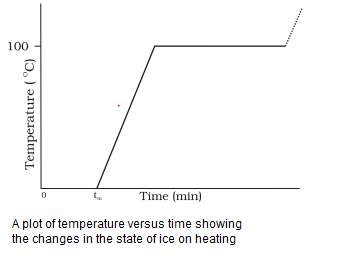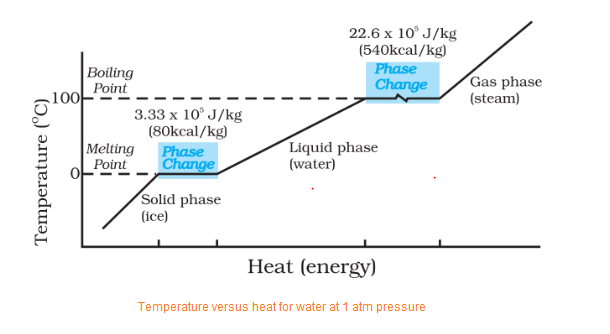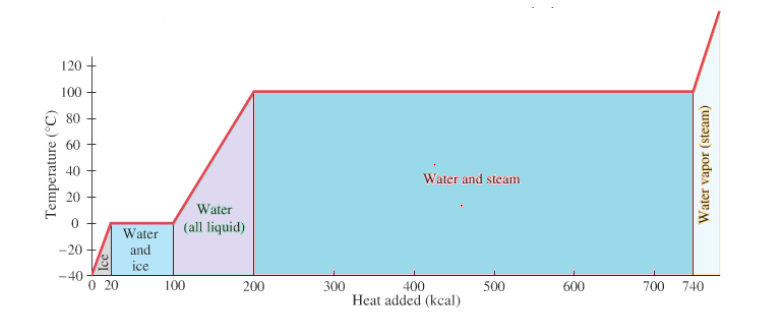# Change of Phases and Latent Heat

## Change of phases:

• There are three phases of matter i.e, Solid, Liquid and Gas.
• Substance for example, H2O exists in solid phase as Ice,in liquid phase as Water and in gas state as Steam.
• Transition from one phase to another phase are accompanied by absorption or liberation of heat and usually by change in volume even at constant T.
• Change of phase from solid to liquid is called melting , from liquid to solid is called fusion and from liquid to gas is called vaporisation
• Once the temperature for phase change is reached( e,g melting or boiling temperature) no further temperature change occurs until all the substance has undergone phase change.

Melting Point:-Temperature at which solid and liquid phase are in thermal equilibrium with each other
Boiling point:-Temperature at which liquid and vapour phase are in thermal equilibrium with each other

• Take some cubes of ice in a beaker. Note the temperature of ice (0 °C). Start heating it slowly on a constant heat source. Note the temperature after every minute. . Draw a graph between temperature and time . You will observe no change in the temperature so long as there is ice in the beaker. In the above process, the temperature of the system does not change even though heat is being continuously supplied. The heat supplied is being utilised in changing the state from solid (ice) to liquid (water).• Change of phase from solid state to Vapour state without passing through liquid state is called sublimation for e.g Dry Ice and Iodine sublimes.

## Latent Heat:

• The amount of heat per unit mass that must be transferred as heat when a sample completely undergoes phase change is called latent Heat of the substance for the process.
• Thus when a sample of mass m completely undergoes phase change,the total energy transferred is
Q=Lm
where,
L- Latent heat and is characteristics of a substance
• Its unit is JKg-1.
• Latent Heat for a solid liquid change is called Latent Heat of Fusion Lf.
• Latent Heat for a liquid gas change is called Latent Heat of Vaporisation Lv.
• The plot of Temperature and heat energy is given belowWe can observe that water requires Latent Heat of Fusion for conversion from ice to liquid at constant temperature 0°C and Latent Heat of Vaporisation for conversion from liquid to gas at constant temperature 100° C
Another graph could be like below## Solved Example

Question 1
When 0.15 kg of ice of 0 °C mixed with 0.30 kg of water at 50 °C in a container, the resulting temperature is 6.7 °C. Calculate the heat of fusion of ice. ($s_{water} = 4186 J kg^{-1} K^{-1}$)
Solution
Heat lost by water = $ms_w \Delta T$
$= (0.30 kg ) (4186 (50.0 - 6.7 )$
$= 54376.14$ J
Heat required to melt ice = $m_iL_f = (0.15 kg) L_f$
Heat required to raise temperature of ice water to final temperature = $m_is_w \Delta T$
$= (0.15 kg) (4186 ) (6.7 - 0 )$
$= 4206.93 J$
Heat lost = heat gained
$54376.14 = (0.15 kg) L_f + 4206.93$
$L_f = 3.34 \times 10^5 J /kg$

Question 2
How much energy does the freezer has to remove from 1.5 kg of water at 20° C to make ice at -10°C? ($s_{water} = 4186 J kg^{-1} K^{-1}$ and $L_f = 3.34 \times 10^5 J /kg$, $s_{ice} = 2100 J kg^{-1} K^{-1}$ )
Solution
Heat Removed = Heat removed from 20° C to 0° C + Latent heat of Fusion + Heat removed from 0° C to -10° C
$= 1.5 \times 4186 \times (20 -0) + 1.5 \times 3.34 \times 10^5 + 1.5 \times 2100 \times (0 - (-10))$
$=125480 + 501000+31500$
$=658 kJ$